## Question 147 Chapter 4 of +2-B – T.S. Grewal 12 Class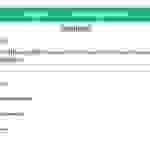Question 147 Chapter 4 of +2-B

Miscellaneous

147. From the following informations, calculate Return on Investment or Return on Capital Employed :

 Rs Share Capital 5,00,000 Reserves and Surplus 2,50,000 Net Fixed Assets 22,50,000 Non-current Trade Investments 2,50,000 Current Assets 11,00,000 10% Long-term Borrowings 20,00,000 Current Liabilities 8,50,000 Long-term Provision Nil

### The solution of Question 147 Chapter 4 of +2-B: –

I

 Net Profit before tax = Rs 6,00,000 Net Profit before interest, tax and dividend = Net Profit before tax + Interest on long-term borrowings = Rs 6,00,000 + Rs 2,00,000 = Rs 8,00,000 Capital Employed = Share Capital + Reserves and Surplus + Long-term borrowings = Rs 5,00,000 + Rs 2,50,000 + Rs 20,00,000 = Rs 27,50,000
 Return on Investment Ratio = Net Profit before interest, tax and dividend X 100 Capital Employed
 Return on Investment Ratio = Rs. 8,00,000 X 100 Rs 27,50,000 = 29.09%

Thanks, Please Like and share with your friends

Comment if you have any question.

Also, Check out the solved question of previous Chapters: –

### Check out T.S. Grewal +2 Book 2020@ Official Website of Sultan Chand PublicationT.S. Grewal’s Analysis of Financial Statements

## Question 146 Chapter 4 of +2-B – T.S. Grewal 12 Class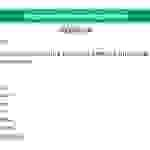Question 146 Chapter 4 of +2-B

Miscellaneous

146. Calculate Current Ratio, Quick Ratio and Debt to Equity Ratio from the
figures given below:

 Particulars Rs Inventory 30,000 Prepaid Expenses 2,000 Other Current Assets 50,000 Current Liabilities 40,000 12% Debentures 30,000 Accumulated Profits 10,000 Equity Share Capital 1,00,000 Non-current Investments 15,000

### The solution of Question 146 Chapter 4 of +2-B: –

I

 Current Assets = Inventory + Prepaid Expenses + Other Current Assets = Rs 30,000 + Rs 2,000 + Rs 50,000 = Rs 82,000 Current Liabilities = Rs 40,000
 Current Ratio = Current Assets Current Liabilities
 Current Ratio = Rs. 82,000 Rs 40,000 = 2.05 : 1

II

 Liquid Assets = Current Assets − Inventory − Prepaid Expenses = Rs 82,000 − Rs 30,000 − Rs 2,000 = Rs 50,000
 Liquid Ratio = Liquid Assets Current Liabilities
 Liquid Ratio = Rs. 50,000 Rs 40,000 = 1.25 : 1

III

 Long term Debts = Rs 30,000 Equity = Accumulated Profits + Equity Share Capital = Rs 10,000 + Rs 1,00,000 = Rs 1,10,000
 Debt Equity Ratio = Debts Equity
 Debt Equity Ratio = Rs. 30,000 Rs 1,10,000 = 0.27 : 1

Thanks, Please Like and share with your friends

Comment if you have any question.

Also, Check out the solved question of previous Chapters: –

### T.S. Grewal’s Double Entry Book Keeping (Vol. II: Accounting for Companies)T.S. Grewal’s Analysis of Financial Statements

## Question 145 Chapter 4 of +2-B – T.S. Grewal 12 Class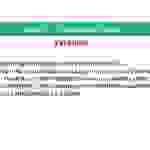Question 145 Chapter 4 of +2-B

Miscellaneous

145. From the following information related to Naveen Ltd.,
calculate (a) Return on Investment and (b) Total Assets to Debt Ratio:
Information: Fixed Assets Rs 75,00,000; Current Assets Rs 40,00,000; Current Liabilities Rs 27,00,000; 12% Debentures Rs 80,00,000 and Net Profit before Interest, Tax and Dividend Rs 14,50,000.

### The solution of Question 145 Chapter 4 of +2-B: –

I

 Net Profit before Interest & Tax = Rs 14,50,000 Capital Employed = Fixed Assets + Current Assets – Current Liabilities = Rs 75,00,000 + Rs 40,00,000 – Rs 27,00,000 = Rs 88,00,000
 Return on Investment Ratio = Net Profit before Interest & Tax X 100 Capital Employed
 Return on Investment Ratio = Rs. 14,50,000 X 100 Rs 88,00,000 = 16.48%

II

 Total Assets = Fixed Assets + Current Assets = Rs 75,00,000 + Rs 40,00,000 = Rs 1,15,00,000 Long term Debts = Rs 80,00,000
 Total Assets to Debts Ratio = Total Assets Long term Debts
 = Rs. 1,15,00,000 Rs 80,00,000 = 1.44 : 1

Thanks, Please Like and share with your friends

Comment if you have any question.

Also, Check out the solved question of previous Chapters: –

### T.S. Grewal’s Double Entry Book Keeping (Vol. II: Accounting for Companies)T.S. Grewal’s Analysis of Financial Statements

## Question 144 Chapter 4 of +2-B – T.S. Grewal 12 Class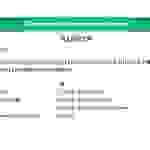Question 144 Chapter 4 of +2-B

Miscellaneous

144. From the following, calculate (a) Debt to Equity Ratio; (b) Total Assets to Debt Ratio; and (c) Proprietary Ratio:

 Rs Rs Equity Share Capital 75,000 Debentures 75,000 Preference Share Capital 25,000 Trade Payable 40,000 General Reserve 45,000 Outstanding Expenses 10,000 Balance in Statement of Profit and Loss 30,000

### The solution of Question 144 Chapter 4 of +2-B: –

I

 Long-term Debts = Rs 75,000 Equity = Equity Share Capital + Preference Share Capital + General Reserve + Balance in Statement of Profit & Loss = Rs 75,000 + Rs 25,000 + Rs 45,000 + Rs 30,000 = Rs 1,75,000
 Debt – Equity Ratio = Long-term Debts Equity
 Gross Profit Ratio = Rs. 75,000 Rs 1,75,000 = 0.43 : 1

II

 Total Assets = Equity Share Capital + Preference Share Capital + General Reserve + Balance in Statement of Profit & Loss + Debentures + Trade Payables + Outstanding Expenses = Rs 75,000 + Rs 25,000 +Rs 45,000 + Rs 30,000 + Rs 75,000 + Rs 40,000 + Rs10,000 = Rs 3,00,000 Long term Debts = Rs 75,000
 Total Assets to Debts Ratio = Total Assets Long term Debts
 = Rs. 3,00,000 Rs 75,000 = 4 : 1

III

 Shareholders’ Funds = Equity Share Capital + Preference Share Capital + General Reserve + Balance in Statement of Profit & Loss = 75,000+25,000+45,000+30,000 = Rs 1,75,000 Total Assets = Equity Share Capital + Preference Share Capital + General Reserve +Balance in Statement of Profit & Loss + Debentures + Trade Payables + Outstanding Expenses = Rs 75,000 + Rs 25,000 + Rs 45,000 + Rs 30,000 + Rs 75,000 + Rs 40,000 + Rs 10,000 = Rs 3,00,000
 Proprietary Ratio = Shareholders’ Funds Total Assets
 = Rs. 1,75,000 Rs 3,00,000 = 0.58 : 1

Thanks, Please Like and share with your friends

Comment if you have any question.

Also, Check out the solved question of previous Chapters: –

### T.S. Grewal’s Double Entry Book Keeping (Vol. II: Accounting for Companies)T.S. Grewal’s Analysis of Financial Statements

## Question 143 Chapter 4 of +2-B – T.S. Grewal 12 Class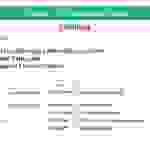Question 143 Chapter 4 of +2-B

Miscellaneous

143. On the basis of the following information calculate:
(i) Debt to Equity Ratio; and
(ii) Working Capital Turnover Ratio.
Information:

 Rs Rs Revenue from Operations (a) Cash Sales 40,00,000 Paid-up Share Capital 17,00,000 (b) Credit Sales 20,00,000 6% Debentures 3,00,000 Cost of Goods Sold 35,00,000 9% Loan from Bank 7,00,000 Other Current Assets 8,00,000 Debentures Redemption Reserve 3,00,000 Current Liabilities 4,00,000 Closing Inventory 1,00,000

### The solution of Question 143 Chapter 4 of +2-B: –

I

 Long-term Debts = 6% Debentures + 9% Loan from Bank = Rs 3,00,000 – Rs 7,00,000 = Rs 10,00,000 Equity = Paid-up Share Capital + Debenture Redemption Reserve = Rs 17,00,000 – Rs 3,00,000 = Rs 20,00,000
 Debt – Equity Ratio = Long-term Debts Equity
 Gross Profit Ratio = Rs. 10,00,000 Rs 20,00,000 = 0.5 : 1

II

 Current Assets = Other Current Assets + Inventory = Rs 8,00,000 + Rs 1,00,000 = Rs 9,00,000 Working Capital = Current Assets − Current Liabilities = Rs 9,00,000 – Rs 4,00,000 = Rs 5,00,000 Net Sales = Cash Sales + Credit sales = Rs 40,00,000 + Rs 20,00,000 = Rs 60,00,000
 Working Capital Turnover Ratio = Net Sales Working Capital
 Operating Ratio = Rs. 60,00,000 Rs 5,00,000 = 12 Times

Thanks, Please Like and share with your friends

Comment if you have any question.

Also, Check out the solved question of previous Chapters: –

### T.S. Grewal’s Double Entry Book Keeping (Vol. II: Accounting for Companies)T.S. Grewal’s Analysis of Financial Statements

## Question 142 Chapter 4 of +2-B – T.S. Grewal 12 Class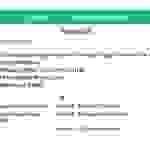Question 142 Chapter 4 of +2-B

Miscellaneous

142. From the information given below, calculate any three of the following ratio:
(i)Gross Profit Ratio;
(ii) Working Capital Turnover Ratio:
(iii)Debt to Equity Ratio; and
(iv) Proprietary Ratio.

 Rs Rs Revenue from Operations Net Sales 5,00,000 Current Liabilities 1,40,000 Cost of Revenue from Operations (Cost of Goods Sold) 3,00,000 Paid-up Share Capital 2,50,000 Current Assets 2,00,000 13% Debentures 1,00,000

### The solution of Question 142 Chapter 4 of +2-B: –

I

 Net Sales = Rs 5,00,000 Cost of Goods Sold = Rs 35,000 Gross Profit = Net Sales − Cost of Goods Sold = Rs 5,00,000 – Rs 3,00,000 = Rs 2,00,000
 Gross Profit Ratio = Gross Profit X 100 Net Sales
 Gross Profit Ratio = Rs 2,00,000 X 100 Rs 5,00,000 = 40%

II

 Net Sales = Rs 1,20,000 Operating Cost = Cost of Goods Sold + Operating Expenses = Rs 60,000 + Rs 40,000 = Rs 1,00,000
 Operating Ratio = Operating Cost X 100 Net Sales
 Operating Ratio = Rs. 1,00,000 X 100 Rs 1,20,000 = 83.33%

III

 Long-term Debts = Rs 1,00,000 Equity = Rs 2,50,000
 Debt – Equity Ratio = Long-term Debts Equity
 Debt – Equity Ratio = Rs. 1,00,000 Rs 2,50,000 = 0.4 : 1

IV

 Total Assets = Total Liabilities Operating Cost = Current Liabilities + Paid-up Share Capital + 13% Debentures = Rs 1,40,000 + Rs 2,50,000 + Rs 1,00,000 = Rs 4,90,000
 Proprietary Ratio = Shareholders’ Fund Total Assets
 Proprietary Ratio = Rs. 2,50,000 Rs 4,90,000 = 0.51 : 1

Thanks, Please Like and share with your friends

Comment if you have any question.

Also, Check out the solved question of previous Chapters: –

### T.S. Grewal’s Double Entry Book Keeping (Vol. II: Accounting for Companies)T.S. Grewal’s Analysis of Financial Statements

## Question 141 Chapter 4 of +2-B – T.S. Grewal 12 Class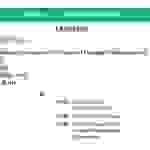Question 141 Chapter 4 of +2-B

Miscellaneous

141Calculate following ratios on the basis of the given information:
(i)Current Ratio;
(ii)Acid Test Ratio;
(iii)Operating Ratio; and
(iv)Gross Profit Ratio.

 Rs Rs Current Assets 70,000 Revenue from Operations Sales 1,20,000 Current Liabilities 35,000 Operating Expenses 40,000 Inventory 30,000 Cost of Goods Sold or Cost of Revenue from Operations 60,000

### The solution of Question 141 Chapter 4 of +2-B: –

I

 Current Assets = Rs 70,000 Current Liabilities = Rs 35,000
 Gross Profit Ratio = Current Assets Current Liabilities
 Gross Profit Ratio = Rs. 70,000 Rs. 70,000 = 2 : 1

II

 Liquid Assets = Current Assets − Inventory = Rs 70,000 − Rs 30,000 = Rs 40,000
 Acid Test Ratio = Liquid Assets Current Liabilities
 Acid Test Ratio = Rs. 40,000 Rs 35,000 = 1.14 : 1

III

 Net Sales = Rs 1,20,000 Operating Cost = Cost of Goods Sold + Operating Expenses = Rs 60,000 + Rs 40,000 = Rs 1,00,000
 Operating Ratio = Operating Cost X 100 Net Sales
 Operating Ratio = Rs. 1,00,000 X 100 Rs 1,20,000 = 83.33%

IV

 Gross Profit = Net Sales − Cost of Goods Sold = Rs 1,20,000 – Rs 60,000 = Rs 60,000 Average Inventory = Rs 15,000
 Gross Profit Ratio = Gross Profit X 100 Net Sales
 Gross Profit Ratio = Rs 60,000 X 100 Rs 1,20,000 = 50%

Balance Sheet: Meaning, Format & Examples

Thanks, Please Like and share with your friends

Comment if you have any question.

Also, Check out the solved question of previous Chapters: –

### T.S. Grewal’s Double Entry Book Keeping (Vol. II: Accounting for Companies)T.S. Grewal’s Analysis of Financial Statements

## Question 140 Chapter 4 of +2-B – T.S. Grewal 12 Class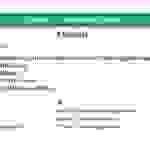Question 140 Chapter 4 of +2-B

Miscellaneous

140. Calculate following ratios on the basis of the following information:
(i)Gross Profit Ratio;
(ii)Current Ratio;
(iii)Acid Test Ratio; and
(iv) Inventory Turnover Ratio.

 Rs Rs Gross Profit 50,000 Revenue from Operations 1,00,000 Inventory 15,000 Trade Receivables 27,500 Cash and Cash Equivalents 17,500 Current Liabilities 40,000

### The solution of Question 140 Chapter 4 of +2-B: –

I

 Gross Profit Ratio = Gross Profit X 100 Revenue from Operations
 Gross Profit Ratio = Rs. 50,000 X 100 Rs 1,00,000 = 50%

II

 Current Ratio = Current Assets Current Liabilities
 Current Assets = Inventory + Cash and Cash Equivalents + Trade Receivables = Rs 60,000 Current Liabilities = Rs 40,000

 Current Ratio = Rs. 60,000 Rs 40,000 = 1.5 : 1

III

 Liquid Ratio = Liquid Assets Current Liabilities
 Liquid Assets = Cash and Cash Equivalents + Trade Receivables = Rs 17,500 + Rs 27,500 = Rs 45,000
 = Rs 45,000 Rs 40,000 = 1.125 : 1

IV

 Inventory Turnover Ratio = Cost of Goods Sold Average Inventory
 Cost of Goods Sold = Revenue from Operations − Gross Profit = Rs 1,00,000 − Rs 50,000 = Rs 50,000 Average Inventory = Rs 15,000
 = Rs 50,000 Rs 15,000 = 3.33 Times

Balance Sheet: Meaning, Format & Examples

Thanks, Please Like and share with your friends

Comment if you have any question.

Also, Check out the solved question of previous Chapters: –

### T.S. Grewal’s Double Entry Book Keeping (Vol. II: Accounting for Companies)T.S. Grewal’s Analysis of Financial Statements

## Question 139 Chapter 4 of +2-B – T.S. Grewal 12 Class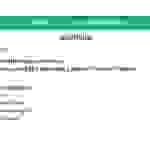Question 139 Chapter 4 of +2-B

Miscellaneous

139. From the following calculate:
(a) Current Ratio; and (b) Working Capital Turnover Ratio.

 Rs i Revenue from Operations 1,50,000 ii Total Assets 1,00,000 iii Shareholders’ Funds 60,000 iv Non-current Liabilities 20,000 v Non-current Assets 50,000

### The solution of Question 139 Chapter 4 of +2-B: –

 Current Assets = Total Assets – Non Current Assets = Rs. 1,00,000 – Rs 50,000 = Rs 50,000 Total Liabilities = Shareholders’ Funds + Non Current Liabilities + Current Liabilities Rs 1,00,000 = Rs. 60,000 + Rs 20,000 + Current Liabilities Current Liabilities = Rs 1,00,000 – Rs 80,000 = Rs 20,000

 Current Ratio = Current Assets Current Liabilities
 Current Ratio = Rs. 50,000 Rs 20,000 = 2.5 : 1

 Working Capital = Current Assets – Current Liabilities = Rs 50,000 − Rs 20,000 = Rs 30,000
 Working Capital Turnover Ratio = Revenue from Operations Working Capital
 = Rs 1,50,000 Rs 30,000 = 5 Times

Balance Sheet: Meaning, Format & Examples

Thanks, Please Like and share with your friends

Comment if you have any question.

Also, Check out the solved question of previous Chapters: –

### T.S. Grewal’s Double Entry Book Keeping (Vol. II: Accounting for Companies)T.S. Grewal’s Analysis of Financial Statements

## Question 138 Chapter 4 of +2-B – T.S. Grewal 12 Class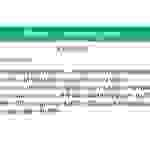Question 138 Chapter 4 of +2-B

Miscellaneous

138. From the following information, calculate Inventory Turnover Ratio;
Operating Ratio and Working Capital Turnover Ratio:
Opening Inventory 28,000; Closing Inventory 22,000; Purchases 46,000;
Revenue from Operations, i.e., Net Sales 80,000; Return 10,000; Carriage
Inwards 4,000; Office Expenses 4,000; Selling and Distribution Expenses
2,000; Working Capital 40,000.

### The solution of Question 138 Chapter 4 of +2-B: –

 Cost of Goods Sold = Opening Inventory + Purchases + Carriage Inwards − Closing Inventory = Rs 28,000 + Rs 46,000 + Rs 4,000 – Rs 22,000 = Rs. 56,000

 Average Inventory = Opening Inventory + Closing Inventory 2
 Current Ratio = Rs. 28,000 + Rs 22,000 2 = Rs 25,000

 Inventory Turnover Ratio = Cost of Goods Sold Average Inventory
 = Rs. 56,000 Rs. 25,000 = 2.24 Times

 Operating Expenses = Office Expenses + Selling and Distribution Expenses = Rs 4,000 − Rs 2,000 = Rs 6,000 Operating Cost = Cost of Goods Sold + Operating Expenses = Rs 56,000 + Rs 62,000 = Rs 62,000
 Operating Ratio = Operating Cost X 100 Net Sales
 Operating Ratio = Rs 62,000 X 100 Rs 80,000 = 77.5%
 Working Capital = Rs 40,000 Net Sales = Rs 80,000
 Working Capital Turnover Ratio = Net Sales Working Capital
 = Rs 80,000 Rs 40,000 = 2 Times

Balance Sheet: Meaning, Format & Examples

Thanks, Please Like and share with your friends

Comment if you have any question.

Also, Check out the solved question of previous Chapters: –

### T.S. Grewal’s Double Entry Book Keeping (Vol. II: Accounting for Companies)T.S. Grewal’s Analysis of Financial Statements